Problem

# The Los Angeles County Metropolitan Transportation Authority has set a bus mechanical reli...

The Los Angeles County Metropolitan Transportation Authority has set a bus mechanical reliability goal of 3,900 bus miles. Bus mechanical reliability is measured specifically as the number of bus miles between mechanical road calls. Suppose a sample of 100 buses resulted in a sample mean of 3,975 bus miles and a sample standard deviation of 275 bus miles.

a. Is there evidence that the population mean bus miles is more than 3,900 bus miles? (Use a 0.05 level of significance.)

b. Determine the p-value and interpret its meaning.

#### Step-by-Step Solution

Solution 1

State the null and alternative hypothesis.

Null hypothesis: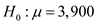That is, the population mean bus miles is 3,900 bus miles.

Alternative hypothesis: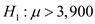That is, the population mean bus miles is more than 3,900 bus miles.

Decision rule:

If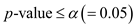, then reject the null hypothesis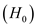.

Use MINITAB to compute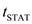.

The formula foris given below:Where,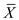is the sample mean,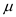is the population mean, S is the sample standard deviation, and n is the sample size.

MINITAB procedure:

Step 1: Choose Stat > Basic Statistics > 1-Sample t.

Step 2: In Summarized data, enter the sample size 100 and mean 3,975.

Step 3: In Standard deviation, enter a value 275.

Step 4: In Perform hypothesis test, enter the test mean 3,900.

Step 5: Check Options, and enter Confidence level as 95.0.

Step 6: Choose greater than in Alternative.

Step 7: Click OK in all dialog boxes.

MINITAB output: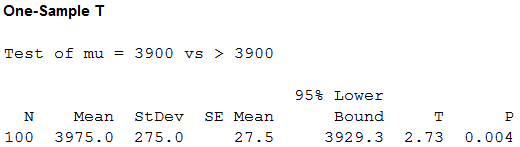From the above MINITAB output, the value ofis 2.73 and the p-value is 0.004.

Interpretation:

Use the significance level,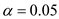Here, p-value is lesser than the level of significance.

That is,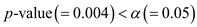.

Therefore, by the rejection rule, it can be concluded that there is an evidence to reject the null hypothesisat. That is, the population mean bus miles is more than 3,900 bus miles.

b.

Find the p -value and interpret:

From the above MINITAB output, the p-value is 0.004. When the population mean bus miles is 3,900 bus miles at 5% significance level then the probability of the test statistic which is greater than 2.73 standard error units away from zero is 0.004.